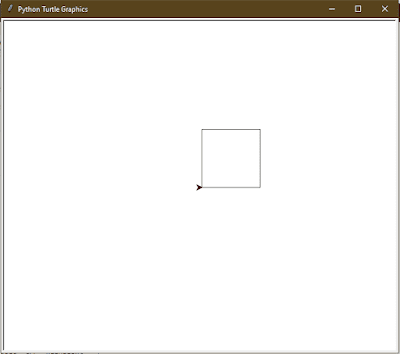# HSSlive: Plus One & Plus Two Notes & Solutions for Kerala State Board

## How to Draw a Square Using Python Turtle, Tkinter, Matplotlib & Without Libraries

How to Draw a Square in Python - There are various methods to create a Square using python libraries like turtle, tkinter and matplotlib. Also, you will learn how to make a Square using python in easy and simple steps. We have also provided the python code for drawing a Square pattern. If you have introductory to intermediate knowledge in Python and statistics, then you can use this article as a one-stop shop for building and plotting Square patterns in Python using libraries from its scientific stack, including NumPy, Matplotlib, Pandas, and Seaborn.

Plotting a Square in python is a simple procedure, and python in its simplest provides multiple easy methods to do so.

## How to Draw a Square using Python?

Given below is the process to draw a Square in python using libraries like Turtle, Tkinter, Matplotib and others.

Four Method to make a Square in Python using multiple libraries:

1. How to draw a Square using python turtle?
2. How to draw a Square using python tkinter?
3. How to draw a Square using python matplotlib?
4. How to draw a Square using python code without library?

## How to Draw a Square using Python Turtle?

Check below step by step process to make a Square using the python turtle library.

Step

Code

# draw square in Python Turtle
import turtle

t = turtle.Turtle()

s = int(input("Enter the length of the side of the Square: "))

# drawing first side
t.forward(s) # Forward turtle by s units
t.left(90) # Turn turtle by 90 degree

# drawing second side
t.forward(s) # Forward turtle by s units
t.left(90) # Turn turtle by 90 degree

# drawing third side
t.forward(s) # Forward turtle by s units
t.left(90) # Turn turtle by 90 degree

# drawing fourth side
t.forward(s) # Forward turtle by s units
t.left(90) # Turn turtle by 90 degree

OutputHow to Draw a Square Using Python Turtle, Tkinter, Matplotlib & Without Libraries

## How to Draw a Square using Python Tkinter?

Check below step by step process to make a Square using the python tkinter library.

Step

Code

Output

## How to Draw a Square using Python Matplotlib?

Check below step by step process to make a Square using the python matplotlib library.

Step

Code

Output

## How to Draw a Square using Python Code without Library?

Step

Code

Output

Note: Also, these codes might not run in an online compiler please use an offline compiler. Programmers can make any changes in the code according to their specific requirement.

## Conclusion on How to Draw a Square using Python

We hope the programs and methods provided above on how to make a Square using python libraries have been helpful to you. If there is any issue in any of the code please let us know in the comments. We will try to resolve it as soon as possible.

Share: